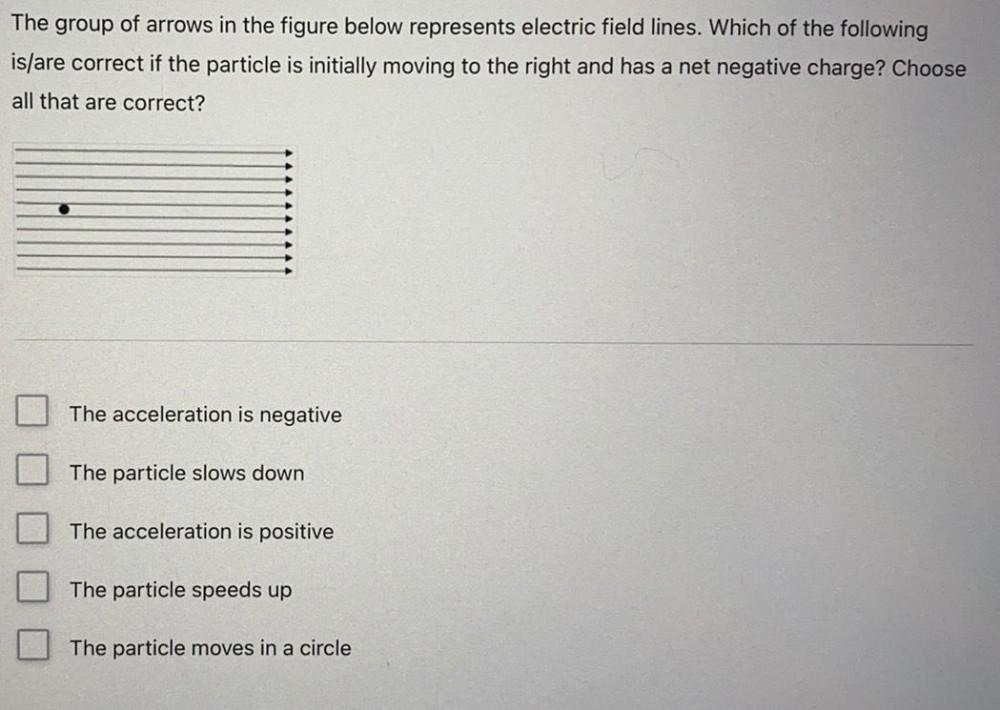Question:

# The group of arrows in the figure below represents electric field lines. Which of the following is/are correct if the particle iThe group of arrows in the figure below represents electric field lines. Which of the following is/are correct if the particle is initially moving to the right and has a net negative charge? Choose all that are correct? The acceleration is negative The particle slows down The acceleration is positive The particle speeds up The particle moves in a circle